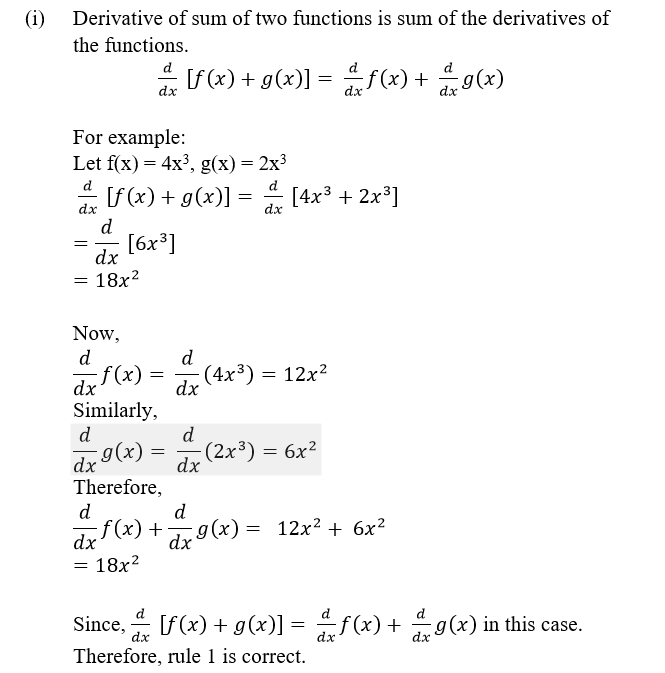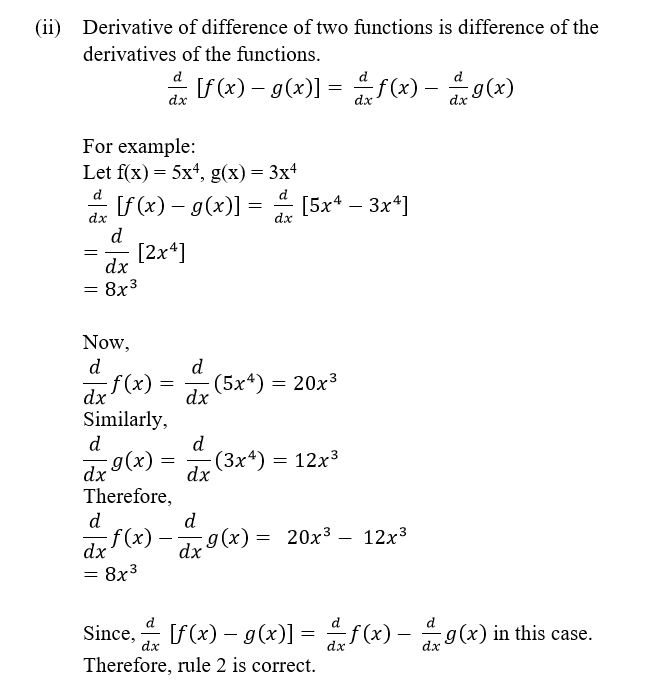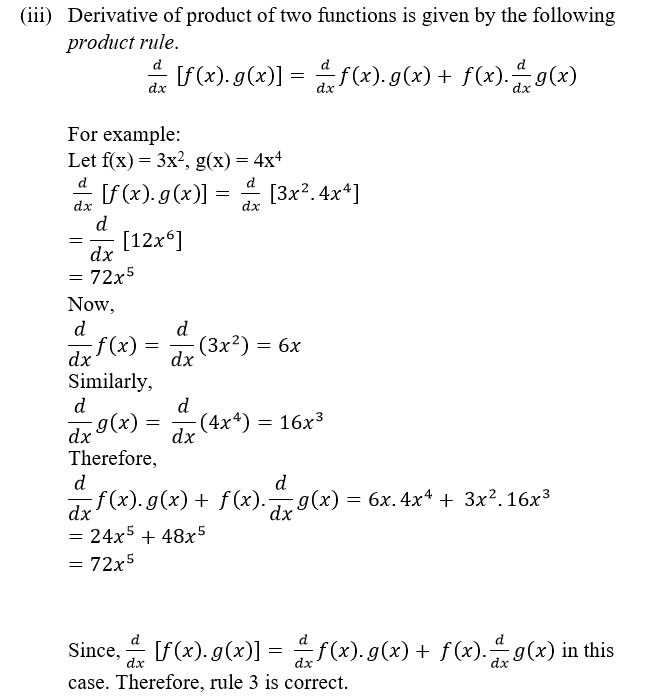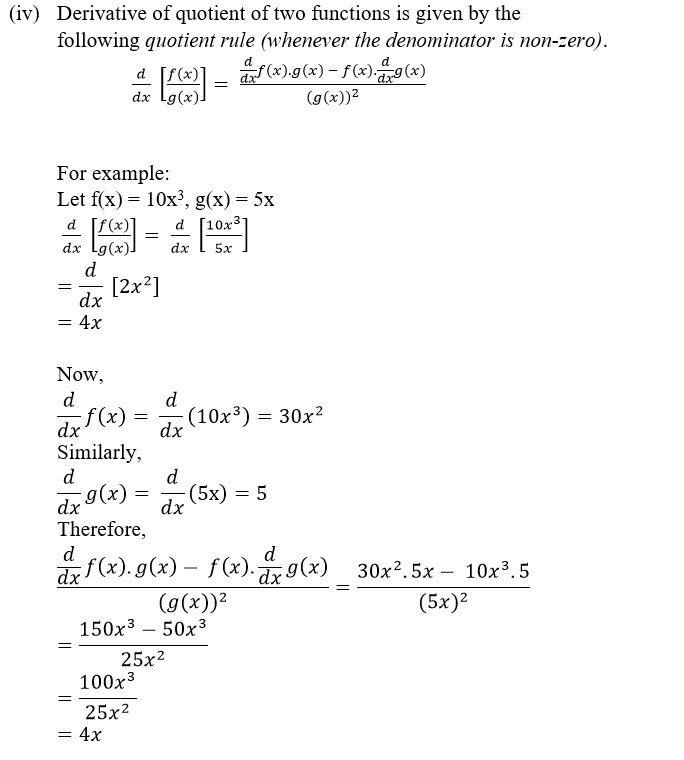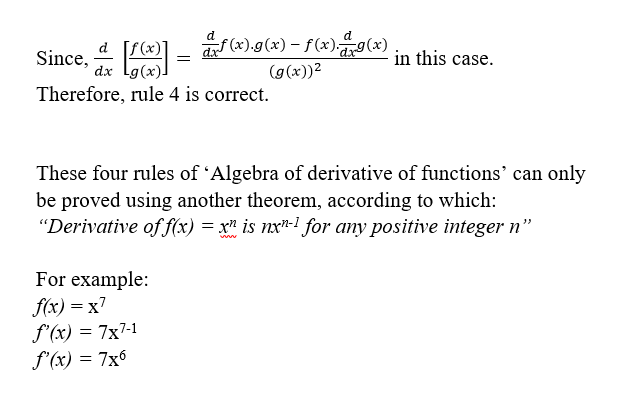Algebra of Derivative of Function
Blog    Algebra of Derivative of Function

## Algebra of Derivative of Function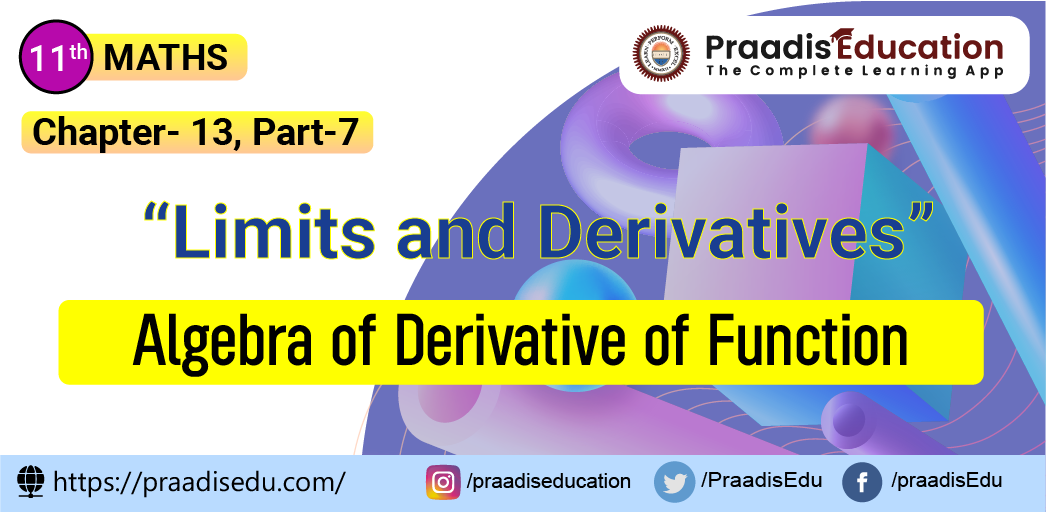Algebra of Derivative of Function is part of the ‘Derivatives’ topic taught in higher classes of Mathematics in school. Algebra of derivative of functions is very similar to the Algebra of limit of functions. There are four different rules of doing Algebra of Derivative of functions.

# Algebra of Derivative of Function

Let f and g be two functions such that their derivatives are defined in a common domain. Then